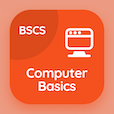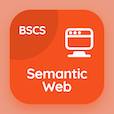Computer Science Courses Online

Chapter 5: Digital Logic Design Practice Tests

Digital Logic Design MCQs - Chapter 5

# Boolean Algebra and Logic Gates Multiple Choice Questions (MCQ Quiz) PDF - 1

The Book Boolean Algebra and Logic Gates Multiple Choice Questions and Answers (MCQs) PDF, Boolean Algebra and Logic Gates quiz answers, test 1 to study online Digital Logic Design Course. Practice Axiomatic Definition of Boolean Algebra MCQs, Boolean Algebra and Logic Gates trivia questions and answers PDF to prepare for job interview. The eBook Boolean Algebra and Logic Gates MCQs App Download: product of maxterms, basic theorems and properties of boolean algebra, boolean functions in dld, basic definition of boolean algebra career test for software engineering online courses.

The Multiple Choice Question (MCQ Quiz): Boolean algebra is also called PDF, Boolean Algebra and Logic Gates App Download (Free) with arithmetic algebra, switching algebra, linear algebra, and algebra choices for BSc computer science. Solve axiomatic definition of boolean algebra quiz questions, download Google eBook (Free Sample) for software engineering online courses.

## Logic Design: Boolean Algebra & Logic Gates MCQs Questions

MCQ: Boolean algebra is also called

A) switching algebra
B) arithmetic algebra
C) linear algebra
D) algebra

MCQ: To perform product of max terms Boolean function must be brought into

A) AND terms
B) OR terms
C) NOT terms
D) NAND terms

MCQ: According to the Boolean algebra absorption law, which of the following is correct?

A) x+xy=x
B) (x+y)=xy
C) xy+y=x
D) x+y=y

MCQ: The expression for Absorption law is given by

A) A + AB = A
B) A + AB = B
C) AB + AA' = A
D) A + B = B + A

MCQ: X*y = y*x is the

A) commutative law
B) inverse property
C) associative law
D) identity element

Download Digital Logic Design Quiz App, Computer Basics MCQ App, and Semantic Web MCQs App to install for Android & iOS devices. These Apps include complete analytics of real time attempts with interactive assessments. Download Play Store & App Store Apps & Enjoy 100% functionality with subscriptions!